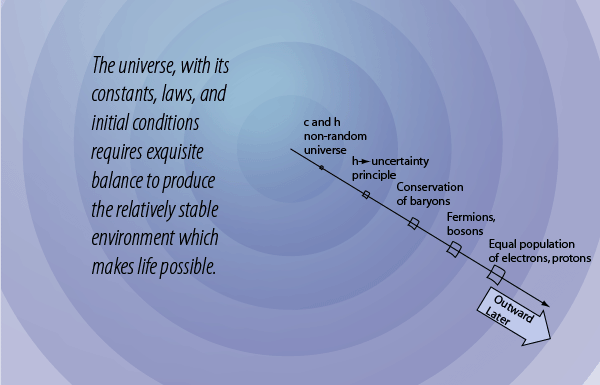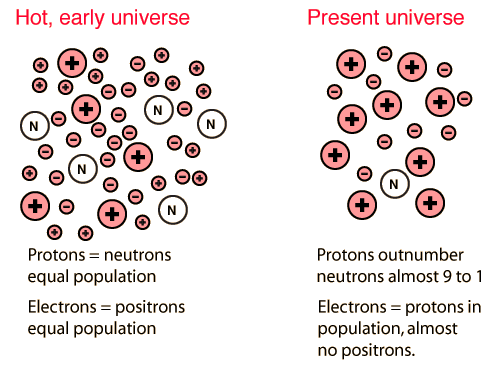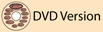# The equality of the number of protons and electronsAs the early universe cooled from the very earliest stages of the hot big bang, the process of the decay of heavier particles produced a large number of protons. This happened under the constraints of the conservation of baryon number . In the current universe, all evidence suggests that the number of electrons in the universe is almost identical to the number of protons, making the universe electrically neutral. If that were not so, the electromagnetic force that is some 1039 times stronger than the gravitational force would dominate the structure of the universe. The evidence suggests that gravity is in fact the dominant force on the large scale. So how is it that the number of electrons is equal to the number of protons?

In the early stages of the big bang the energy available was far above the threshold for electron-positron pair-production, so it is presumed that there would be an equilibrium state with large numbers of electrons and positrons in essentially equal concentration. As the early universe expanded and cooled so that the available energy was below the pair-production threshold, electrons and positrons would have annihilated each other leaving none of either. Yet we observe a large number of electrons in the current universe and no positrons except those being produce in radioactive decay and nuclear interactions. So there had to be some asymmetry which left us with a remnant population of electrons, and just the right number of electrons to give us an electrically neutral universe where gravity is dominant. This classic problem is often called the matter-antimatter problem, and we have some tentative suggestions about how the asymmetry came about. In any case, the process of producing the same number of electrons and protons is one of the fine tuning processes in the production of our universe.

 The balance of the strong and electromagnetic forces
Index

 HyperPhysicsR Nave
Go Back